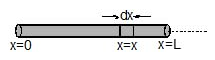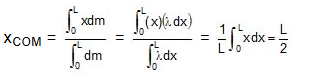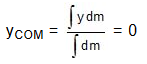Suppose a rod of mass M and length L is lying along the x-axis with its one end at x = 0 and the other at x = L.

Mass per unit length of the rod l = M/L

Hence, dm, (the mass of the element dx situated at x = x is) = l dx
The coordinates of the element dx are (x, 0, 0). Therefore, x-coordinate of COM of the rod will beThe y-coordinate of COM isSimilarly, zCOM = 0

i.e., the coordinates of COM of the rod are ( 0, 0), i.e., it lies at the centre of the rod.

#### 1 COMMENT

1.Sujeet Kumar Sharma

Best method